Refer to our Texas Go Math Grade 2 Answer Key Pdf to score good marks in the exams. Test yourself by practicing the problems from Texas Go Math Grade 2 Lesson 7.3 Answer Key Write Equations to Represent Addition.

Explore

Draw to show how you found the answer.FOR THE TEACHER • Read the following problem and have children choose their own methods for solving. There are 15 children on the bus. Then 9 more children get on the bus. How many children are on the bus now?Explanation:
There are 15 children on the bus.
Then 9 more children get on the bus.
24 children are on the bus now

Math Talk
Mathematical Processes

Explain how you found the number of children on the bus.
We have added the number of children in the bus
and who get on the bus
15 + 9 = 24
so, there are total 24 children in the bus

Model and Draw

You can write a number sentence to show a problem.
Sandy has 16 pencils. Nancy has 13 pencils. How many pencils do the two girls have?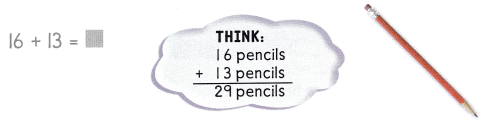The two girls have _________ pencils.
The two girls have 29 pencils.
16 + 13 = 29
number of pencils sandy has = 16
number of pencils Nancy has = 13

Share and Show

Write a number sentence for the problem. Use a ☐ for the missing number. Then solve.

Question 1.
Carl sees 25 melons at the store. 15 are small and the rest are large. How many melons are large?___________ melons
10 melons
Explanation:
Carl sees 25 melons at the store.
15 are small and the rest are large.
25 – 15 = 10
15 + 10 = 25
10 melons are large

Question 2.
83 people went to a movie on Thursday. 53 of them were children and the rest were adults. How many adults were at the movie?
Explanation:
83 people went to a movie on Thursday.
53 of them were children and the rest were adults.
83 – 53 = 30
53 + 30 = 83
30 adults were at the movie

Problem Solving

Write a number sentence for the problem. Use a ☐ for the missing number. Then solve.

Question 3.
Chris and his friends went to the zoo. In the Reptile House, they saw 9 snakes and 19 lizards. How many reptiles did they see?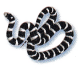__________ reptiles
28 reptiles
Explanation:
Chris and his friends went to the zoo.
In the Reptile House,
they saw 9 snakes and 19 lizards.
9 + 19 = 28
28 reptiles that they see.

Solve. Write or draw to explain.

Question 4.
H.O.T. Multi-Step Braden’s class went to the park. They saw 26 oak trees and 19 maple trees. They also saw 13 cardinals and 35 blue jays. Compare the total number of trees and the total number of birds that the class saw.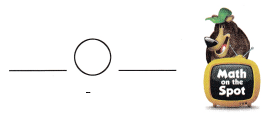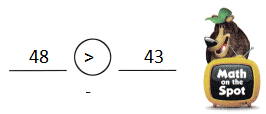Explanation:
26 oak trees and 19 maple trees.
trees = 26 + 19 = 45
13 cardinals and 35 blue jays.
13 + 35 = 48 birds
48 – 45 = 3
48 > 43
birds are greater than trees

Question 5.
H.O.T. Amy needs about 70 paper clips. Without adding, circle 2 boxes that would be close to the amount that she needs.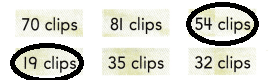Explanation:
54 + 19 = 73
this is 3 more than 70
35 + 32 = 67
this is 3 less than 70

Question 6.
Apply There are 43 whales in the first group. There are 38 whales in the second group. How many whales are in the two groups?
(A) 81
(B) 78
(C) 71
Explanation:
There are 43 whales in the first group.
There are 38 whales in the second group.
43 + 38 = 81
81 whales are in the two groups

Question 7.
There are 43 fish in one group and 38 fish in another group. How many fish are there?
(A) 75
(B) 88
(C) 81
Explanation:
There are 43 fish in one group
and 38 fish in another group.
43 + 38 = 81
81  fish are there

Question 8.
Reasoning In April, Robert counted 49 boats in the lake. Robert counted 47 boats in May. How many boats did Robert count in the two months?
(A) 96
(B) 86
(C) 99
Explanation:
In April, Robert counted 49 boats in the lake.
Robert counted 47 boats in May.
49 + 47 = 96
96 boats that Robert count in the two months

Question 9.
TEXAS Test Prep Mr. Walton baked 54 cookies last week. He baked 38 cookies this week. How many cookies did he bake in the two weeks?(A) 16
(B) 92
(C) 82
Explanation:
Mr. Walton baked 54 cookies last week.
He baked 38 cookies this week.
54 + 38 = 92
92 cookies that he bake in the two weeks

TAKE HOME ACTIVITY • Have your child explain how he or she solved one of the exercises in this lesson.